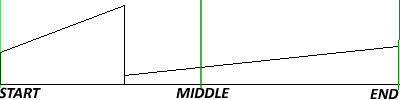# Searching For a Number in Shifted Sorted Array within O(log(n)) time

Run into the algorithm problem long time ago. Now post my answer here. A sorted array, say: {1,2,3,4,5,6,7,8,9,10,11,12}, do right rotate through carry unknown times, and then it might become: {6,7,8,9,10,11,12,1,2,3,4,5}. Now we need get the index of a given number, say 4, from the array within O(log(n)) time. Apparently a 8-year-old can get it done with O(n) time.

We can think of it this way: take the middle element of array, if target is found, fine; if not, and then array become two parts, one is sorted array, the other is shifted sorted array. As illustrated as below diagram:If the target falls into the sorted array half, we can simple do a binary search; otherwise, repeat this operation in the other half in recursive way. You can see this is divide-and-conquer algorithm. Obviously this is O(log(n)).

Code

 // // A typical binary search implementation // int _BinarySearch(unsigned int ShiftedArray[], unsigned int start, unsigned int end, unsigned int target) {     // Not found     if( start == end && ShiftedArray[start] != target) {        return -1;     }     unsigned int middle = start + (end - start)/2;     if(target == ShiftedArray[middle])     {        return middle;     } else if (target > ShiftedArray[middle]) {        return _BinarySearch(ShiftedArray, middle + 1, end, target);     } else {        return _BinarySearch(ShiftedArray, start, middle - 1, target);     } } // // Select a given number from shifted array. // ShiftedArray is something like = {6,7,8,9,10,11,12,1,2,3,4,5} // If found, return index of the number; if not, reutrn -1 // Require log(N) // int SearchShiftedArray(unsigned int ShiftedArray[], unsigned int start, unsigned int end, unsigned int target) {     // Start meets end     if( start == end && ShiftedArray[start] != target) {        return -1;     }     unsigned int middle = start + (end - start)/2;     if(target == ShiftedArray[middle])     {        return middle;     } else if(ShiftedArray[middle] < ShiftedArray[start]) { // Right half is sorted linearly        if((target > ShiftedArray[middle]) && (target <= ShiftedArray[end])) {            return _BinarySearch(ShiftedArray, middle + 1, end, target);        } else {            return SearchShiftedArray(ShiftedArray, start, middle-1, target);        }     } else { // Left half is sorted linearly        if((target >= ShiftedArray[start]) && (target < ShiftedArray[middle])) {            return _BinarySearch(ShiftedArray, start, middle - 1, target);        } else {            return SearchShiftedArray(ShiftedArray, middle + 1, end, target);        }     } }

Test cases

 Positive: {6,7,8,9,10,11,12,1,2,3,4,5}, target = 3, target = 8 Negative: {6,7,8,9,10,11,12,1,2,3,4,5}, target = 0, target = 13 Boundary: {6,7,8,9,10,11,12,1,2,3,4,5}, target = 6, target = 5 Exceptional: {…max}, target = max

One more interesting thing is the statement that “only about 10 percent of the professional programmers implemented binary search correctly. ” Do you know why? Check this.